# 3rd Class Power Engineering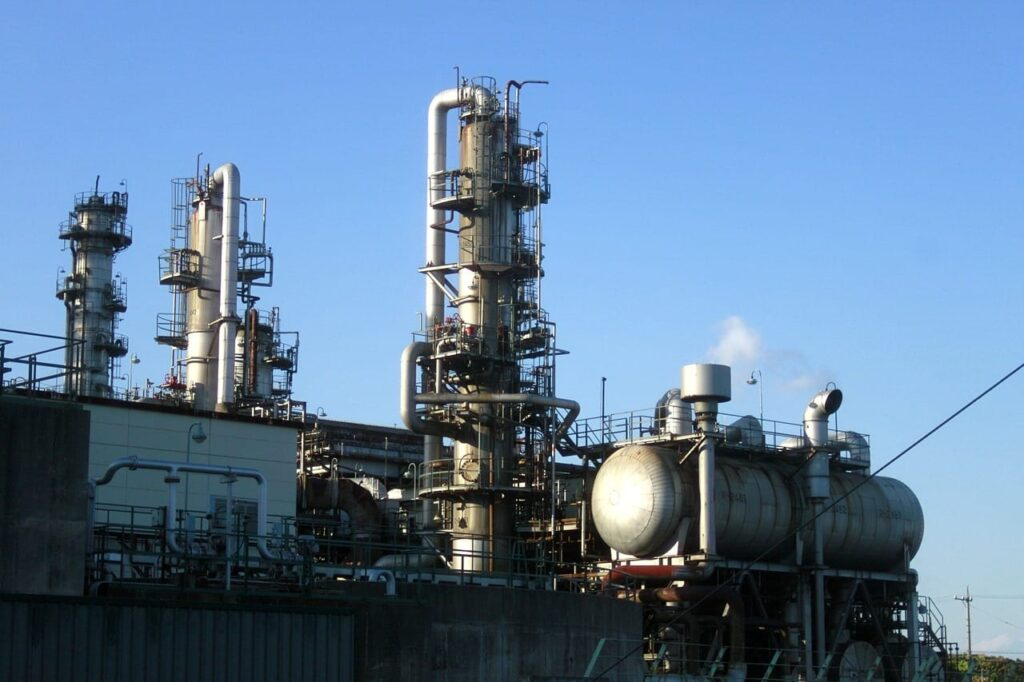## How To: Calculate The Thickness Of An Unstayed Full Hemispherical Head

When completing ASME code calculations involving cylindrical components it is possible during examinations you will be required to know how to calculate the thickness of an unstayed full hemispherical head. I recommend before working on questions involving full hemispherical heads, you read over the material and complete the practice questions regarding How To: Determine The Thickness Of …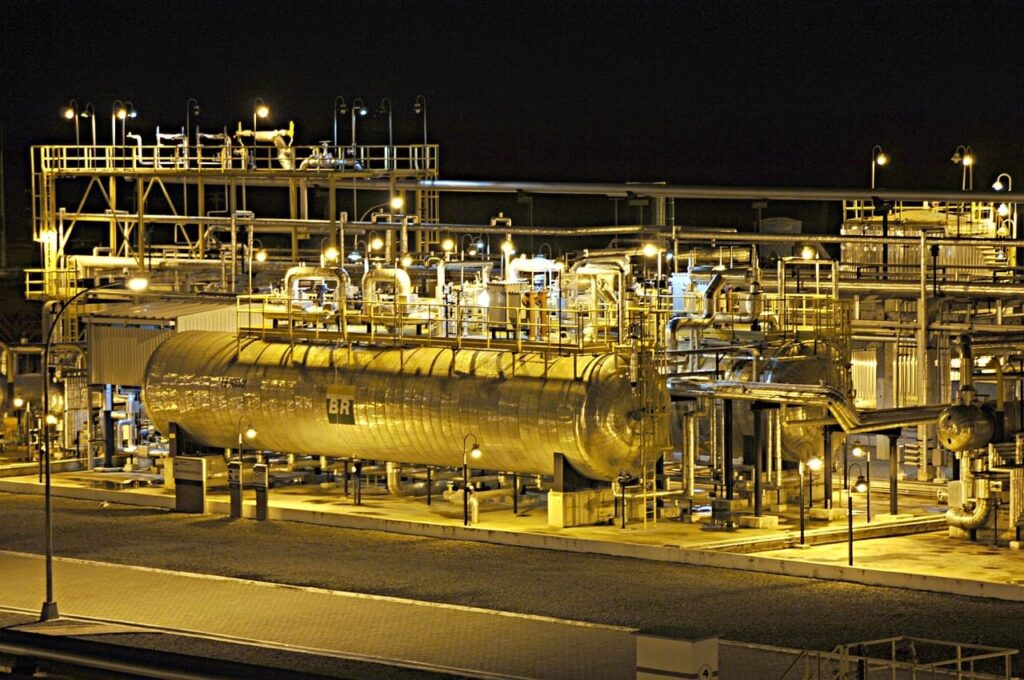## How To: Calculate The Thickness Of A Blank, Unstayed Dished Head

The topic we’re going to cover in this post involves determining the minimum required thickness of a dished head, when it is a blank unstayed dished head with pressure on the concave side, and a segment of a sphere. We will review the formula, provide where to find it, define each of the variables, and then provide …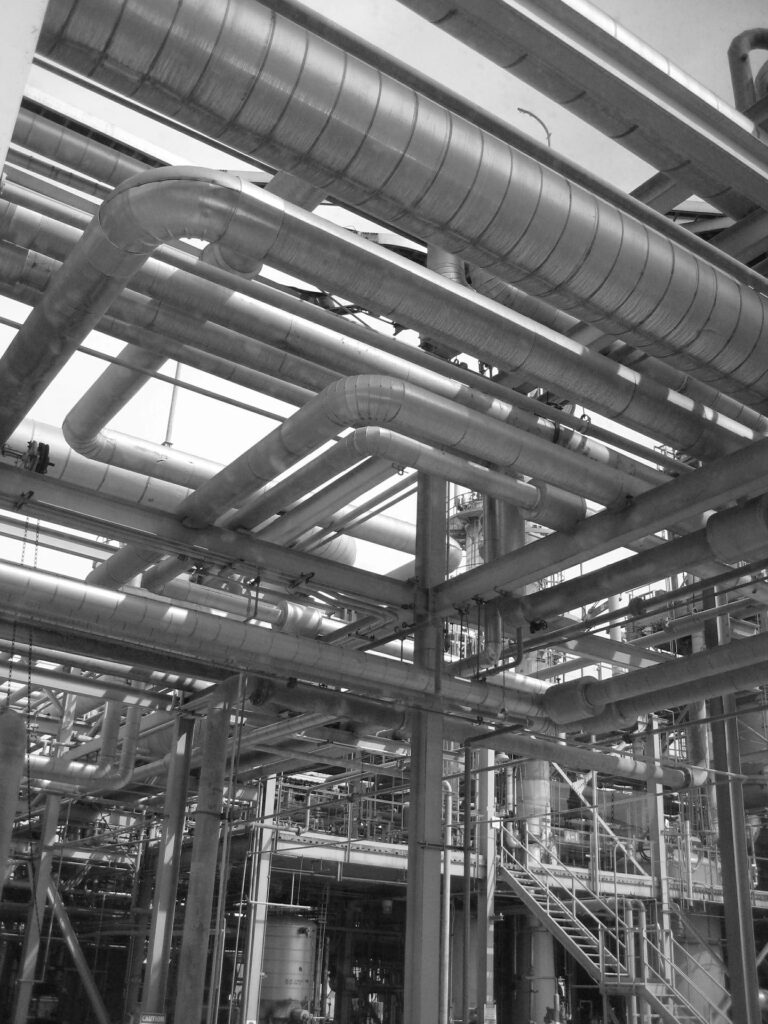## How To: Calculate The Maximum Allowable Working Pressure Of A Header

When completing ASME code calculations involving cylindrical components you will be required to know how to calculate the maximum allowable working pressure of a header. All page numbers referenced below are from the 2007 ASME Boiler & Pressure Vessel Code. Formula To calculate the maximum allowable working pressure of a header during different operating conditions you …## How To: Calculate The Minimum Required Thickness Of A Header

Throughout your 3rd Class Power Engineering studies you’ll be required to complete ASME code calculations involving cylindrical components. More specifically, you’ll be required to know how to calculate the minimum required thickness of a header on your provincial examination.   All page numbers referenced below are from the 2007 ASME Boiler & Pressure Vessel Code. Formula To …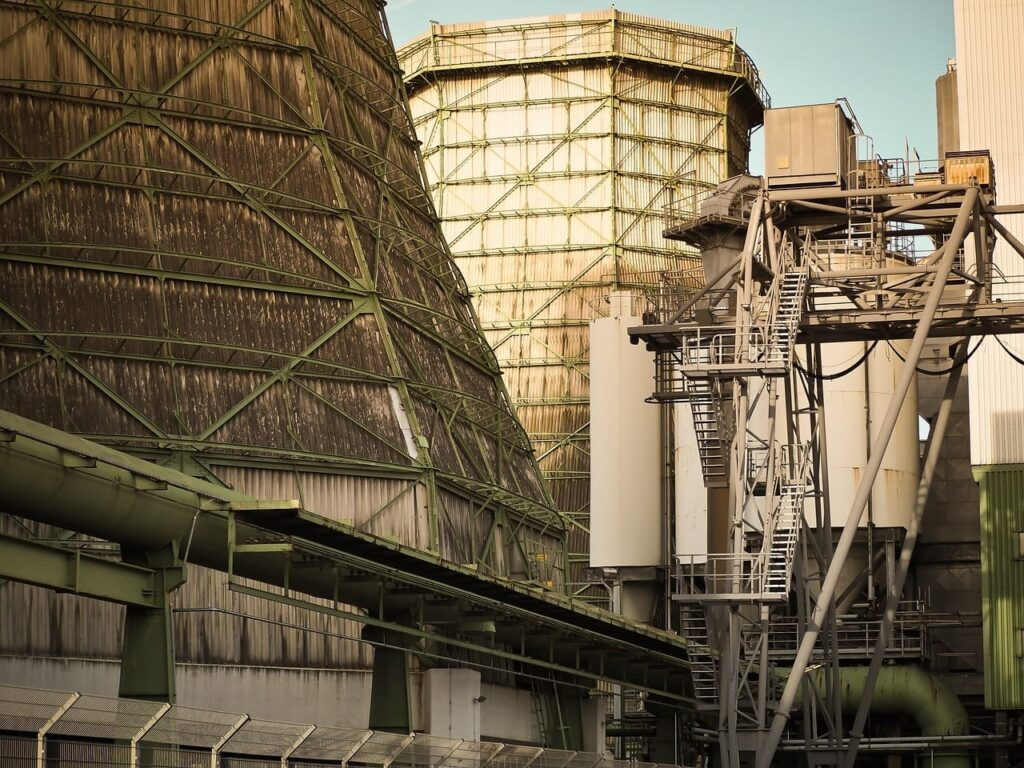## How To: Calculate The Maximum Allowable Working Pressure Of A Boiler Drum

While completing ASME code calculations involving cylindrical components for 3rd Class Power Engineering you’ll be required to know how to calculate the maximum allowable working pressure of a boiler drum.  The referenced page numbers below are from the 2007 ASME Boiler & Pressure Vessel Code. Formula To calculate the maximum allowable working pressure of a …## How To: Calculate The Minimum Required Thickness Of A Boiler Drum

In 3rd Class Power Engineering ASME code calculations you’ll be required to know how to calculate the minimum required thickness of a boiler drum. The information provided below helps explain the material covered in:   All referenced page numbers below are from the 2007 ASME Boiler & Pressure Vessel Code. Formula To calculate the minimum …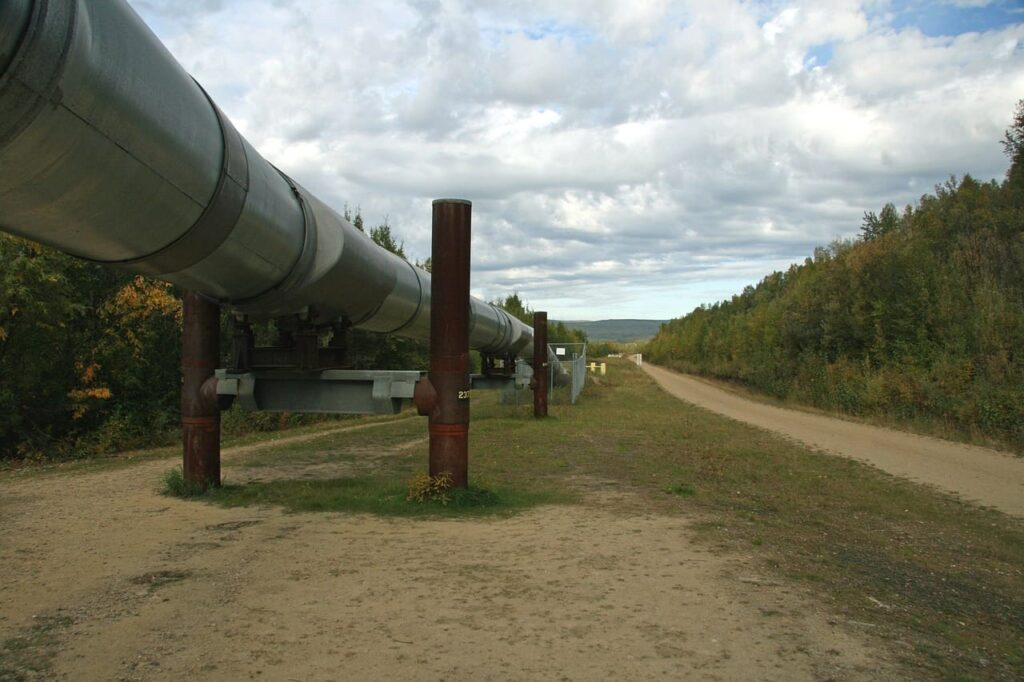## How To: Calculate The Maximum Allowable Working Pressure Of Piping

While preparing for your 3A2 exam you’ll be required to complete ASME code calculations involving how to calculate the maximum allowable working pressure of piping. If you read through the material below. Complete the practice questions provided. You’ll have a much better understanding of the information covered within:  All page numbers referenced below are from …## How To: Calculate The Minimum Required Thickness Of Piping

While completing 3rd Class ASME code calculations involving cylindrical components you’ll be required to know how to calculate the minimum required thickness of piping. The objective of this post is to provide you with a clear understanding of how to accomplish this and explain the material covered in:   All page numbers referenced below are …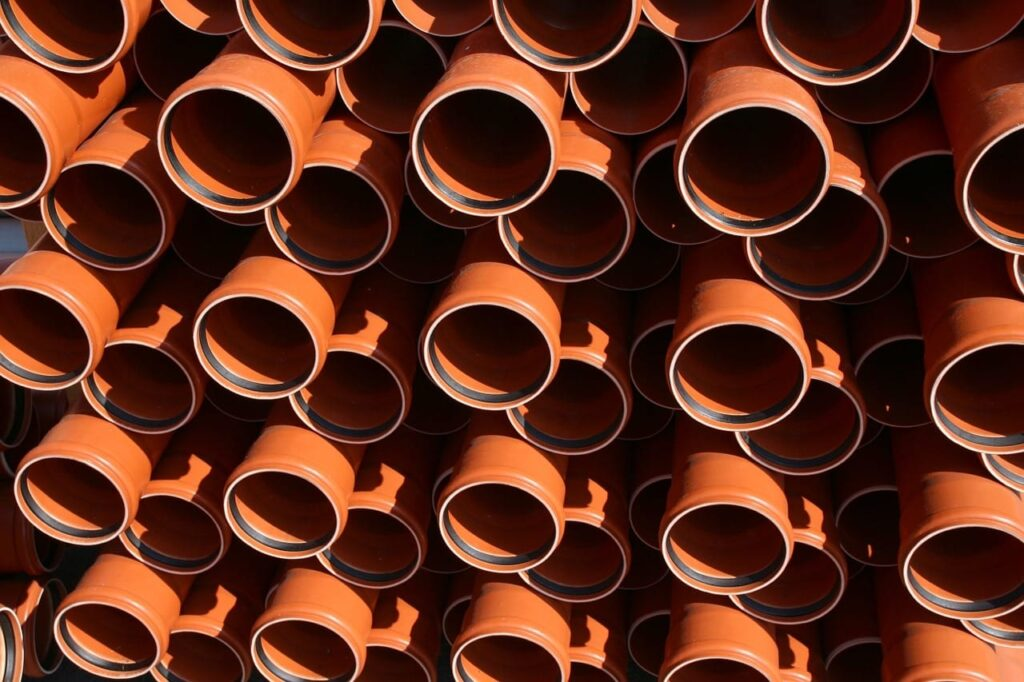## How To: Calculate The Maximum Allowable Working Pressure Of Tubing

Throughout your 3rd Class Power Engineering studies you’ll be required to complete ASME code calculations involving cylindrical components. One of the code calculation you’ll be required to know how to complete is How To Calculate The Maximum Allowable Working Pressure Of Tubing. The tubing you’re required to determine the maximum allowable working pressure of, could be …## How To: Calculate The Minimum Required Wall Thickness For Tubing

In ASME code calculations involving cylindrical components for 3rd Class Power Engineering you’ll be required to know How To Calculate The Minimum Required Wall Thickness For Tubing. The tubing you may be required to determine the minimum thickness of could be but not limited to superheater tubes or water tube boiler tubes. This post helps explain …## How Does A Transformer Work?

The objective of this post is to answer the question How Does A Transformer Work? By the end of this post you’ll have a better understanding of the following topics: General overview of transformers Principle of operation Transformer application Single-phase transformers Three-phase transformers Transformer calculations Power transmission losses Core & Shell transformers Transformer cooling Autotransformers …